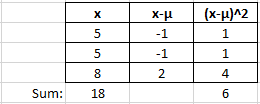## Datasets

Consider a set of three values: 5, 5, 8. What is the Mean, Variance, and Median for the dataset?

Hint
The mean is the average of all the data points. The median is the value separating the higher half from the lower half of the data set.
$$variance=\sigma^{2}=(\frac{1}{N})\sum_{i=1}^{N}(x_{1}-\mu)^{2}$$$$$\mu=18/3=6=mean$$$
The median is 5, which is the value that separates the higher half from the lower half of the dataset.
$$variance=\sigma^{2}=(\frac{1}{N})\sum_{i=1}^{N}(x_{1}-\mu)^{2}$$$$$variance=\frac{1}{3}(6)=2$$$
$$mean=6$$$$$median=5$$$
$$variance = 2$$\$